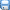## 2015版七年级数学上册提技能+题组训练 3.1《建立一元一次方程模型》 湘教版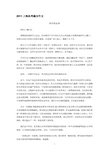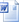资料类别:  数学/同步 所属版本:  浙教版 所属地区:  全国 上传时间:  2015/11/5 下载次数:  18 次 资料类型: 上传人:  zkWS****@163.com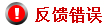### 资料概述与简介

3.1 建立一元一次方程模型 提技能·题组训练 一元一次方程的有关概念 1.下列方程为一元一次方程的是　(　　) A.x+5=y+4　　　　　 B.=1 C.x2-x=1 D.x=0 【解析】选D.选项A有两个未知数;选项B中的不是整式;选项C中未知数的最高次数是2,故A,B,C都不是一元一次方程,选项D符合一元一次方程的定义. 【变式训练】已知下列方程:①x-2=;②0.3x=1;③=5;④x2-4x=3;⑤5x=0; ⑥x+2y=0,其中是一元一次方程的有　(　　) A.2个　　　 B.3个　 　　 C.4个　 　　 D.5个 【解析】选B.按照一元一次方程的定义,②③⑤为一元一次方程,故共有3个. 2.一元一次方程2x=4的解是　(　　) A.x=1 B.x=2 C.x=3 D.x=4 【解析】选B.把四个选项依次代入方程检验,将A,C,D代入时,等式两边的值都不相等,而当x=2时,等式两边的值相等. 3.(2014·合肥模拟)若关于x的方程2x-a=x-2的解为x=3,则字母a的值为　 (　　) A.-5 B.5 C.-7 D.7 【解析】选B.由方程2x-a=x-2的解为x=3, 将x=3代入方程得:2×3-a=3-2, 即6-a=1, 解得:a=5. 4.下列各式:①2x-5=3;②5+4=9;③4y-5;④3m-5n=7;⑤3x2-2x=7;⑥x+3>4;⑦x+2≠3,是等式的是　　　　　,是方程的是　　　　. 【解析】含有等号的式子为等式,等式有①②④⑤;含有未知数的等式叫做方程,方程有①④⑤. 答案:①②④⑤　①④⑤ 5.(2014·安庆模拟)已知mxn-1-8=0是关于x的一元一次方程,则m　　　　　　　,n　　　　　　　. 【解析】因为x的次数为1,所以n-1=1,即n=2;方程中必须有含有未知数x的项,所以m≠0. 答案:≠0　=2 【易错提醒】一元一次方程的条件是“只含一个未知数,未知数的次数是1,等号两边都是整式”,三个条件缺一不可.此题不能只考虑n的范围而忽略了m不能为0这个条件. 6.请写出一个解为x=-2的一元一次方程　　　　　　　　. 【解析】先写一个含有-2的等式,然后把-2用x代替即可得到一元一次方程. 答案:x+1=-1(答案不唯一) 7.已知y=1是方程my=y+2的解,求m2-3m+1的值. 【解析】因为y=1是方程my=y+2的解, 所以m=1+2,故m=3, 当m=3时,m2-3m+1=9-3×3+1=1. 列一元一次方程 1.小悦买书需用48元钱,付款时恰好用了1元和5元的纸币共12张.设所用的1元纸币为x张,根据题意,下面所列方程正确的是　(　　) A.x+5(12-x)=48 B.x+5(x-12)=48 C.x+12(x-5)=48 D.5x+(12-x)=48 【解析】选A.1元纸币为x张,那么5元纸币有(12-x)张,所以x+5(12-x)=48. 2.甲乙两数的和为10,并且甲比乙大2,求甲、乙两数.下面所列方程正确的是　 (　　) A.设乙数为x,则x+2=10 B.设乙数为x,则(x-2)+x=10 C.设甲数为x,则(x+2)+x=10 D.设乙数为x,则(x+2)+x=10 【解析】选D.设乙数为x,则甲数为x+2,故(x+2)+x=10. 【变式训练】x的8倍加上4与x的9倍相等,则所列方程为　　　　. 【解析】x的8倍加上4用式子表示为8x+4,x的9倍用式子表示为9x,所以8x+4=9x. 答案:8x+4=9x 3.(2014·甘肃阿阳实验质检)某班把1400元奖学金按照两种奖项奖给22名学生,其中一等奖每人200元,二等奖每人50元,设获得一等奖的学生人数为x人,其中列方程不正确的是　(　　) A.200x+50(22-x)=1400 B.1400-200x=50(22-x) C.=22-x D.50x+200(22-x)=1400 【解析】选D.获得一等奖的学生人数为x人,则获得二等奖的人数为(22-x)人,一等奖奖金共200x元,二等奖奖金共50(22-x)元,根据相等关系“一等奖奖金+二等奖奖金=1400元”,列方程为200x+50(22-x)=1400;根据相等关系“1400元-一等奖奖金=二等奖奖金”,列方程为1400-200x=50(22-x);根据相等关系“(1400元-一等奖奖金)÷二等奖每人的奖金=获二等奖的人数”,列方程为=22-x.选项D不正确. 4.爸爸今年37岁,是儿子年龄的3倍还多1岁,设儿子x岁,列方程为　　　　. 【解析】因为儿子x岁,所以爸爸(3x+1)岁,所以3x+1=37. 答案:3x+1=37 【变式训练】今年父亲32岁,儿子5岁,再过几年父亲的年龄是儿子的4倍?(只列方程) 【解析】设再过x年父亲的年龄是儿子的4倍,再过x年后,父亲的年龄变成(32+x)岁,儿子的年龄变成(5+x)岁,列方程为32+x=4(5+x). 5.七年级(1)班部分同学计划一起租车秋游,租车费人均15元;后来又有4名同学加入,总租车费不变,结果人均少花3元,设原来有x名学生,可列方程为　　　　. 【解析】原来总租车费为15x,加入4名同学后的总租车费为(15-3)(x+4),故方程为(15-3)(x+4)=15x. 答案:(15-3)(x+4)=15x 6.在一次植树活动中,甲班植树的株数比乙班多20%,乙班植树的株数比甲班的一半多10株.设乙班植树x株. (1)列两个不同的含x的代数式,分别表示甲班植树的株数. (2)根据题意列出含未知数x的方程. (3)检验乙班、甲班植树的株数是不是分别为25株和35株. 【解析】(1)根据甲班植树的株数比乙班多20%,得甲班植树的株数为(1+20%)x;根据乙班植树的株数比甲班的一半多10株,得甲班植树的株数为2(x-10). (2)(1+20%)x=2(x-10). (3)把x=25分别代入方程的左边和右边,得 左边=(1+20%)×25=30, 右边=2×(25-10)=30. 因为左边=右边, 所以25是方程(1+20%)x=2(x-10)的解. 这就是说乙班植树的株数是25株,从上面检验过程可得甲班植树的株数是30株,而不是35株. 【错在哪？】作业错例 课堂实拍 已知方程(a-4)x|a|-3+2=0是关于x的一元一次方程,求a的值. (1)找错:第_________步出现错误. (2)纠错: ________________________________________ 答案: (1)④ (2)又因为a-4≠0,所以a≠4,所以a=-4. 初中学习网，资料共分享！我们负责传递知识！www.czxxw.com

## 更多>>其他相关资源### 资料ID：

/ /

…下载本资料需要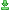下载次